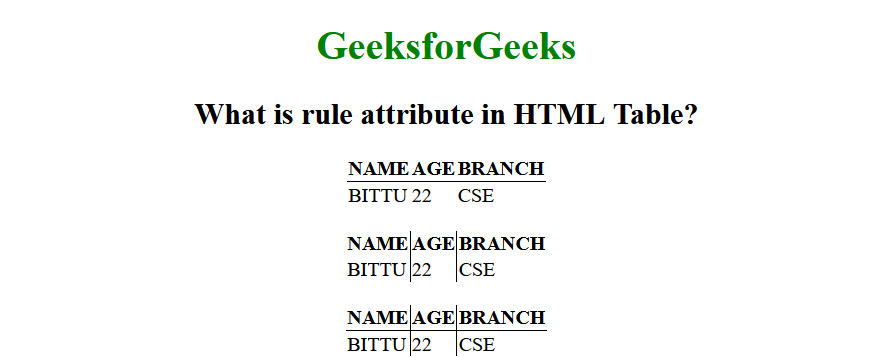GFG App
Open AppBrowser
Continue

# What is the rule attribute in HTML Table ?

This is an HTML attribute, that is used to specify which parts of the inside borders should be visible. The HTML rule attribute is applicable on table tags. The <table> rules attribute is not supported by HTML 5.

Syntax:

`<table rules="value">`

Attributes: This attribute accepts 5 values all are mentioned and described below.

• none: It does not create any lines.
• groups: It creates lines between row and column groups.
• rows: It creates a line between the rows.
• cols: It creates lines between the columns.
• all: It creates lines between the rows and columns.

Example: The below example illustrates the rule attribute in HTML.

## HTML

 ` ` `<``html``> ` ` `  `<``head``> ` `    ``<``style``> ` `        ``h1 { ` `            ``color: green; ` `        ``} ` `    `` ` ` ` ` `  `<``body``> ` `    ``<``center``> ` `    ``<``h1``>GeeksforGeeks ` ` `  `    ``<``h2``>What is rule attribute in HTML Table? ` ` `  `    ``<``table` `rules``=``"rows"``> ` `        ``<``tr``> ` `            ``<``th``>NAME ` `            ``<``th``>AGE ` `            ``<``th``>BRANCH ` `        `` ` `        ``<``tr``> ` `            ``<``td``>BITTU ` `            ``<``td``>22 ` `            ``<``td``>CSE ` `        `` ` `    `` ` `    ``<``br``> ` `    ``<``table` `rules``=``"cols"``> ` `        ``<``tr``> ` `            ``<``th``>NAME ` `            ``<``th``>AGE ` `            ``<``th``>BRANCH ` `        `` ` `        ``<``tr``> ` `            ``<``td``>BITTU ` `            ``<``td``>22 ` `            ``<``td``>CSE ` `        `` ` `    `` ` `    ``<``br``> ` `    ``<``table` `rules``=``"all"``> ` `        ``<``tr``> ` `            ``<``th``>NAME ` `            ``<``th``>AGE ` `            ``<``th``>BRANCH ` `        `` ` `        ``<``tr``> ` `            ``<``td``>BITTU ` `            ``<``td``>22 ` `            ``<``td``>CSE ` `        `` ` `    `` ` `    `` ` ` ` ` `  ` `

Output:HTML rule attribute in HTML Table

My Personal Notes arrow_drop_up